Courses
Courses for Kids
Free study material
Free LIVE classes
More

# Characteristics of an Image by Plane, Concave and Convex Mirror for JEE## What are the Characteristics of an Image?

Last updated date: 28th Mar 2023
Total views: 78.9k
Views today: 1.55k

A mirror image is nothing but a reflection of the object placed in front of the mirror. Interestingly, the images formed have certain characteristics. For example, an image formed is subject to left-right inversion, alphabets may be reversed (however some of them remain the same), the top and bottom of an image remain the same, and so on. When we study image characteristics, we are essentially learning about how an image behaves when it is passed through a plane mirror, a concave mirror, and a convex mirror.

## Characteristics of Image Formed by Plane Mirror

An image is formed at a location where all the light from the object appears to diverge. A person looking in the same direction as the mirror is likely to see the image or replica of an object placed in front of the mirror. Keeping this in mind, let us list four characteristics of an image formed by a plane mirror. Imagine the image of an object when placed in front of a plane mirror.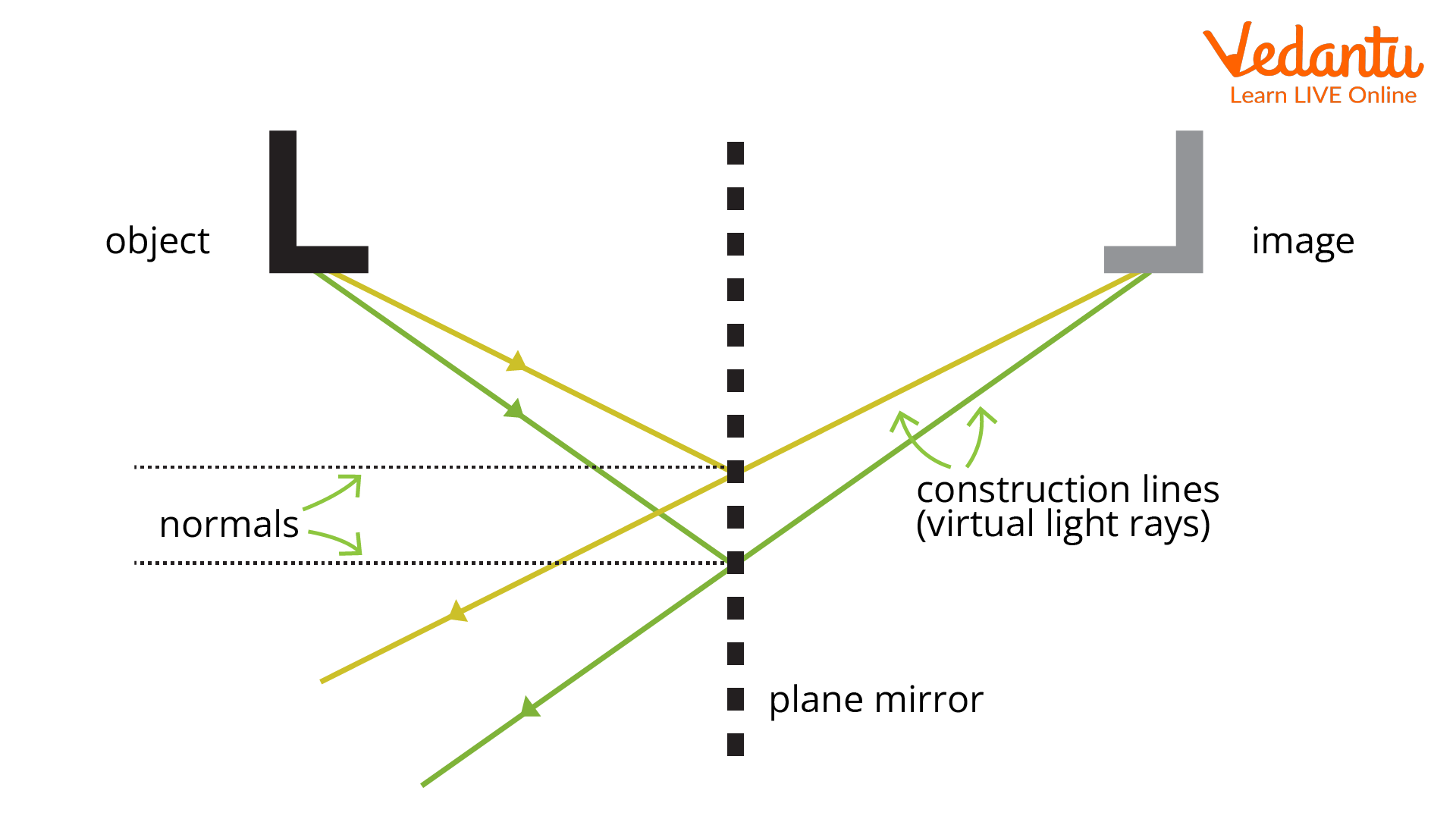Image formed by a plane mirror

The image formed when placed in front of a plane mirror is:

1. Virtual and erect

2. At the same distance as that of the object

3. Of the same size as the object

4. Laterally inverted

All of the characteristics listed above can also be considered as the fundamental characteristics of an image formed by a plane mirror. So what is a laterally inverted image? Laterally inverted images are formed when the orientation of the image formed changes. For example, if you stand in front of a plane mirror holding a book in your left hand but in the mirror, it would seem as if you're holding the book in the right hand. Interestingly, the inversion is only sideways and not top to bottom.

## Characteristics of Image Formed by Concave Mirror

First, let us understand what a concave mirror is. A mirror can have a flat surface or a curved surface. Mirrors with a flat surface are called plane mirrors, while mirrors with a curved surface are either concave or convex. So how can we differentiate between concave and convex mirrors?

Convex mirrors have the inner side of them painted, whereas concave mirrors have the outside surface painted. Thus, for convex mirrors, the outer surface becomes the reflected surface; however, in a concave mirror, the inner side becomes the reflecting surface. Now, let us consider an object placed in front of a concave mirror.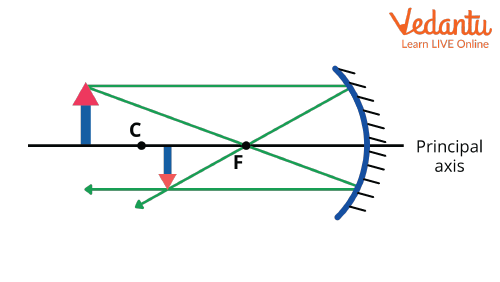Image formed by a concave mirror

The object here is placed behind the centre of curvature C whereas the image is formed between the centre of curvature and focus (F). It must also be stated here that the object here can be placed at different points. For example, the object could be placed at the centre of curvature, between focus and centre of curvature, at focus, or at infinity.

Based on the position of the object, the image formed can be magnified, diminished, erect, inverted, real, or virtual. Thus, the characteristics of images formed by a concave mirror are as follows:

• When an object is placed very close to the mirror, a magnified image is formed. The image formed is also erect and virtual.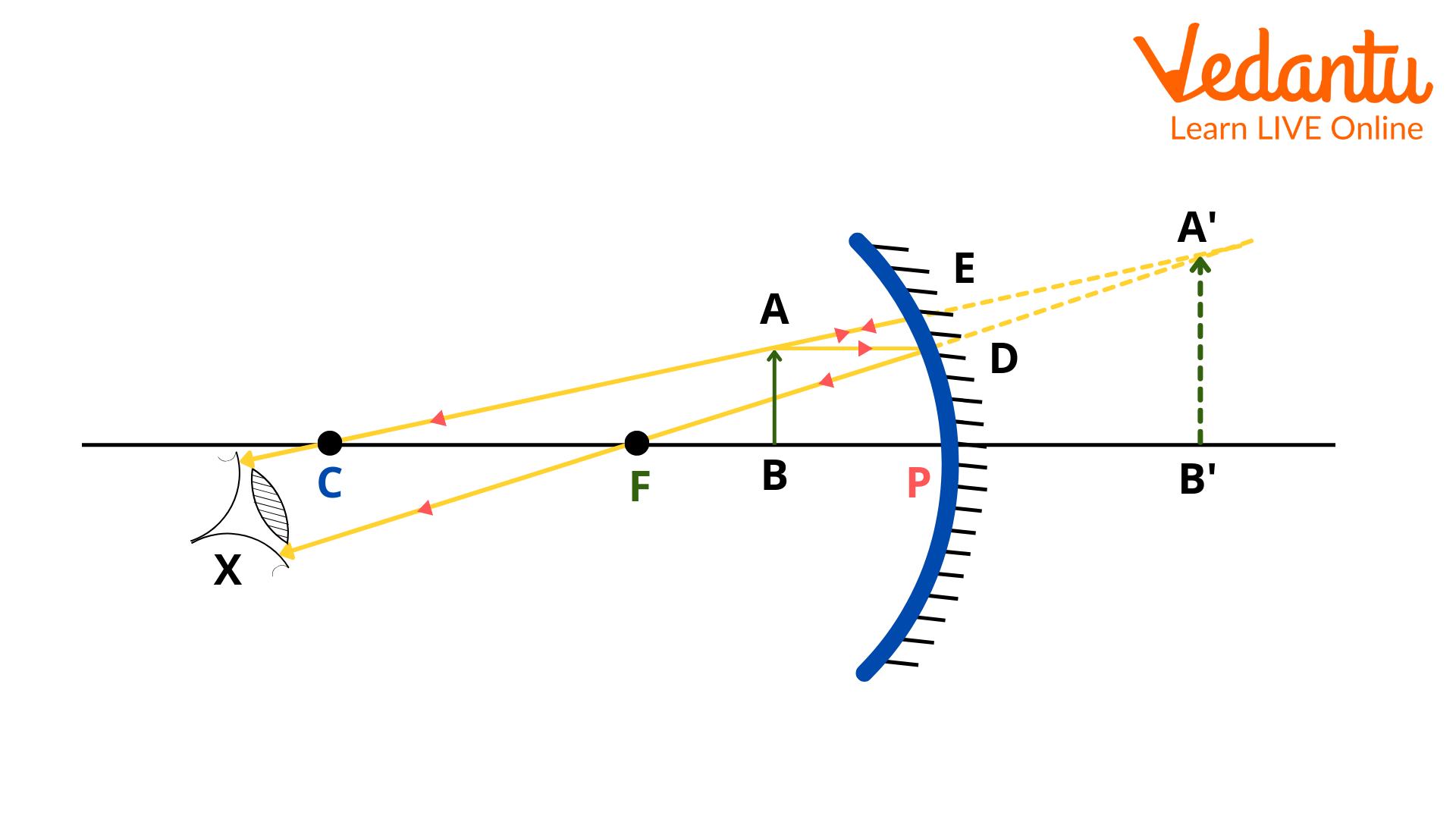Image of an object placed close to the concave mirror

Here C, F, and P are the centre of curvature, focus, and the pole, respectively.

• Secondly, if we place the object far away from the mirror, the image formed is real, inverted, diminished, or small in size.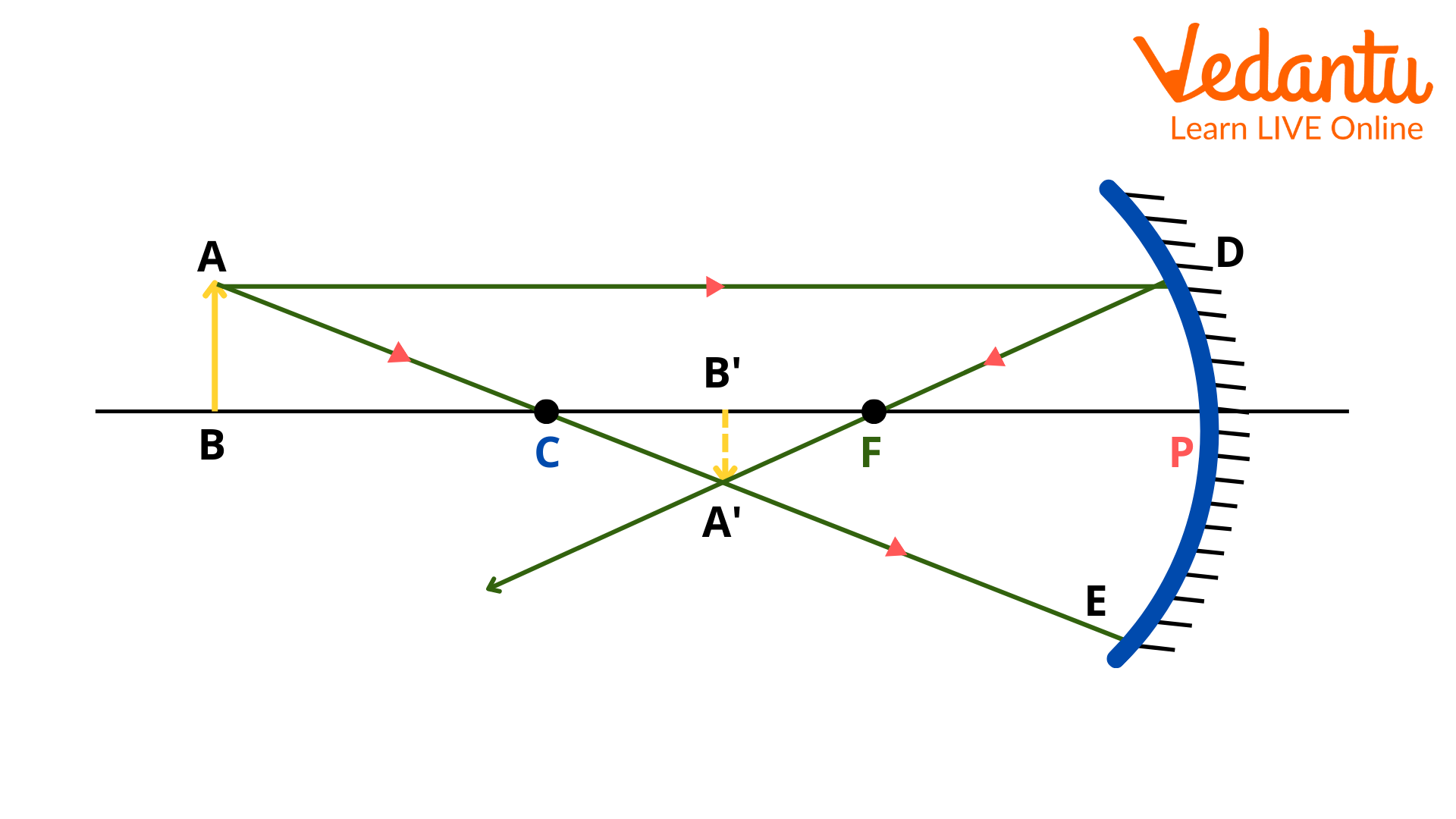Image of an object placed far away from the concave mirror

• Thirdly, when the object is placed at C.

When the object is placed at C, subsequently the image formed is also at C. The image formed in this case is real and of the same size as the object.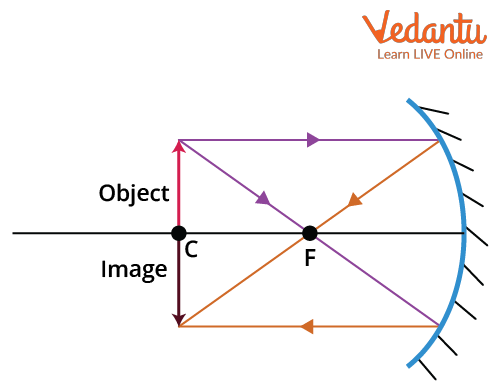Image of an object when placed at C

• Lastly, when the object is placed between C and F. When an object is placed between C and F, the image formed is behind C and is larger than the object.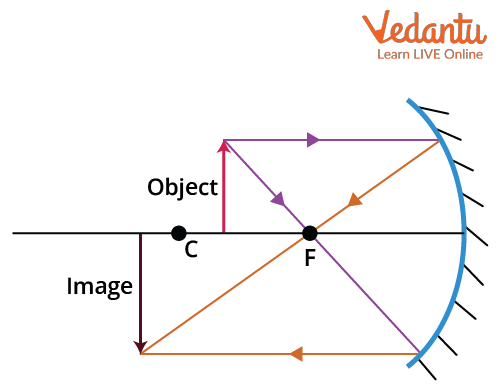Image of an object when placed between C and F

Here, C, F, and P are the centre of curvature, focus, and the pole, respectively. Additionally, concave mirrors are also known as converging mirrors.

## Characteristics of Image Formed by Convex Mirror

First, let us understand what a convex mirror is. As discussed earlier, in convex mirrors, the inner side is painted, and the outer surface is the reflecting surface. Consider an object placed in front of a convex mirror.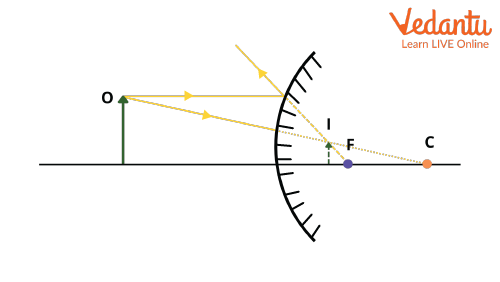Image formed by a convex mirror

Convex mirrors, which are also referred to as diverging mirrors, have the characteristic that they always produce diminished, virtual, and erect images irrespective of the positioning of the object in front of the mirror.

## Conclusion

Mirror images are an important concept in optics. Mirror images can be real or virtual. Depending on the type of surface of the mirror, the mirrors are categorised into the plane and spherical mirrors, respectively. As there are different categories of mirrors, the characteristics of the mirrors also change accordingly. It is important to understand these characteristics of mirrors as they are utilised in many real-life applications.

For example, the rear-view mirror of a car is convex as it forms diminished, erect, and virtual images. Convex mirrors also provide a wider field of view which is why they can be perfectly used in cars. With a myriad of applications in real life, it is necessary to study the characteristics of images for different mirror surfaces.

## FAQs on Characteristics of an Image by Plane, Concave and Convex Mirror for JEE

1. What are real and virtual images?

We talk about images being real and virtual, but what does this actually mean? As seen from above, in a plane mirror, we get virtual images. Virtual images are nothing but images formed when the light does not converge but “seems to converge”. Virtual images can also be said to be formed in locations where light does not actually reach. Real images, on the other hand, are formed by curved or spherical mirrors. Real images are formed on the same side of the object. Also, for real images, the rays converge, whereas for virtual images they diverge.

2. What are ray diagrams?

A ray diagram is nothing but a pictorial representation of how light travels when it strikes the surface of a mirror and how it bounces back. In optics, ray diagrams are used to trace the path of light when an object is placed in front of a mirror and to form an image. Ray diagrams are denoted as line segments with an arrowhead. The arrowhead denotes the direction in which the light travels. Ray diagrams are nothing but the incident and reflected rays. Look at the figure below: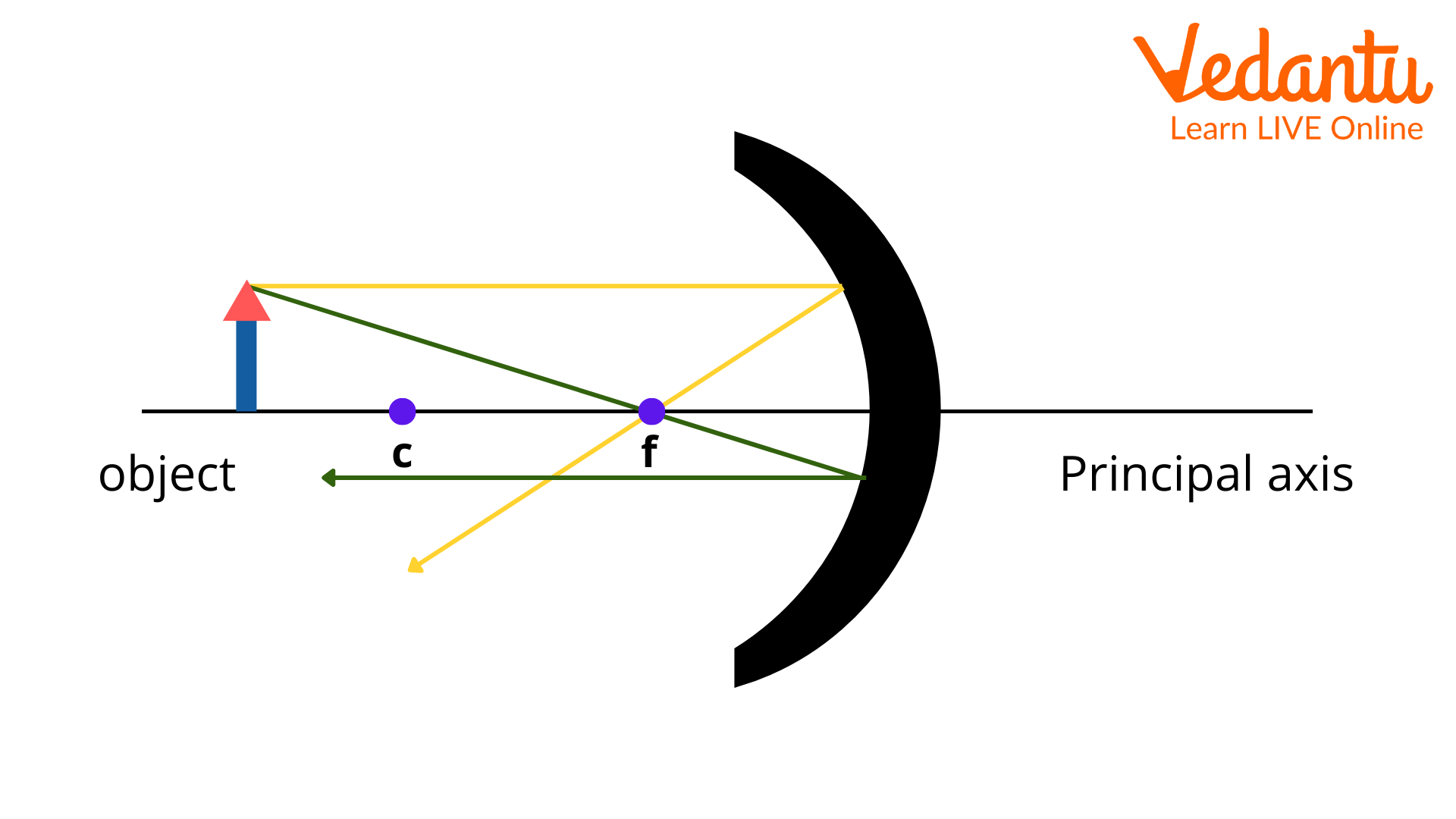Ray Diagram